GeeksforGeeks App
Open AppBrowser
Continue

# Working with excel files using Pandas

Excel sheets are very instinctive and user-friendly, which makes them ideal for manipulating large datasets even for less technical folks. If you are looking for places to learn to manipulate and automate stuff in excel files using Python, look no more. You are at the right place.

## Python Pandas With Excel Sheet

In this article, you will learn how to use Pandas to work with Excel spreadsheets. At the end of the article, you will have the knowledge of:

• Necessary modules are needed for this and how to set them up in your system.
• Reading data from excel files into pandas using Python.
• Exploring the data from excel files in Pandas.
• Using functions to manipulate and reshape the data in Pandas.

### Installation

To install Pandas in Anaconda, we can use the following command in Anaconda Terminal:

`conda install pandas`

To install Pandas in regular Python (Non-Anaconda), we can use the following command in the command prompt:

`pip install pandas`

### Getting Started

First of all, we need to import the Pandas module which can be done by running the command: Pandas

## Python3

 `import` `pandas as pds`

Input File: Let’s suppose the excel file looks like this

Sheet 1: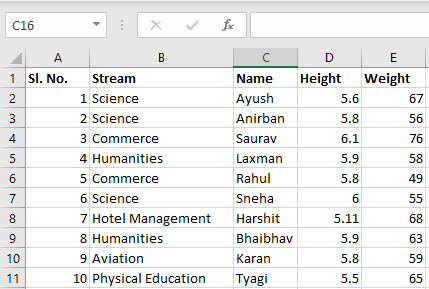Sheet 2: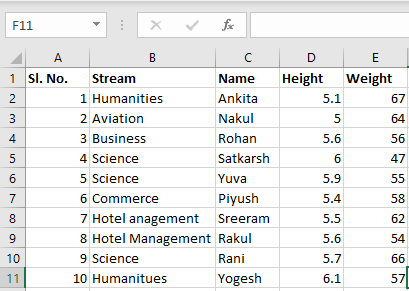Now we can import the excel file using the read_excel function in Pandas. The second statement reads the data from excel and stores it into a pandas Data Frame which is represented by the variable newData. If there are multiple sheets in the excel workbook, the command will import data of the first sheet. To make a data frame with all the sheets in the workbook, the easiest method is to create different data frames separately and then concatenate them. The read_excel method takes argument sheet_name and index_col where we can specify the sheet of which the data frame should be made of and index_col specifies the title column, as is shown below:

## Python3

 `file` `=``(``'path_of_excel_file'``)``newData ``=` `pds.read_excel(``file``)``newData`

Output: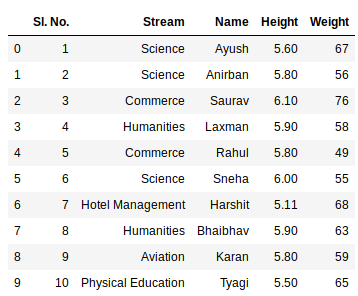Example:

The third statement concatenates both sheets. Now to check the whole data frame, we can simply run the following command:

## Python3

 `sheet1 ``=` `pds.read_excel(``file``,``                        ``sheet_name ``=` `0``,``                        ``index_col ``=` `0``)` `sheet2 ``=` `pds.read_excel(``file``,``                        ``sheet_name ``=` `1``,``                        ``index_col ``=` `0``)` `newData ``=` `pds.concat([sheet1, sheet2])` `newData`

Output: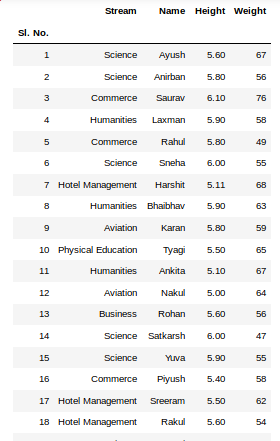To view 5 columns from the top and from the bottom of the data frame, we can run the command. This head() and tail() method also take arguments as numbers for the number of columns to show.

## Python3

 `newData.head()``newData.tail()`

Output: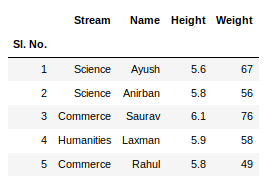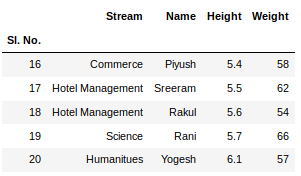The shape() method can be used to view the number of rows and columns in the data frame as follows:

## Python3

 `newData.shape`

Output: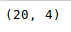If any column contains numerical data, we can sort that column using the sort_values() method in pandas as follows:

## Python3

 `sorted_column ``=` `newData.sort_values([``'Height'``], ascending ``=` `False``)`

Now, let’s suppose we want the top 5 values of the sorted column, we can use the head() method here:

## Python3

 `sorted_column[``'Height'``].head(``5``)`

Output: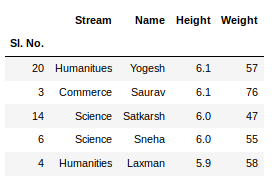We can do that with any numerical column of the data frame as shown below:

## Python3

 `newData[``'Weight'``].head()`

Output: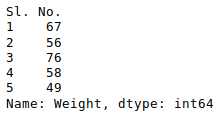Now, suppose our data is mostly numerical. We can get the statistical information like mean, max, min, etc. about the data frame using the describe() method as shown below:

## Python3

 `newData.describe()`

Output: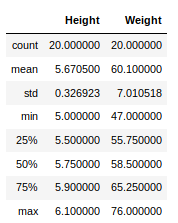This can also be done separately for all the numerical columns using the following command:

## Python3

 `newData[``'Weight'``].mean()`

Output: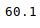Other statistical information can also be calculated using the respective methods. Like in excel, formulas can also be applied and calculated columns can be created as follows:

## Python3

 `newData[``'calculated_column'``] ``=``                ``newData[“Height”] ``+` `newData[“Weight”]``newData[``'calculated_column'``].head()`

Output: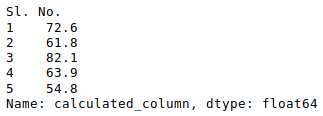After operating on the data in the data frame, we can export the data back to an excel file using the method to_excel. For this we need to specify an output excel file where the transformed data is to be written, as shown below:

## Python3

 `newData.to_excel(``'Output File.xlsx'``)`

Output: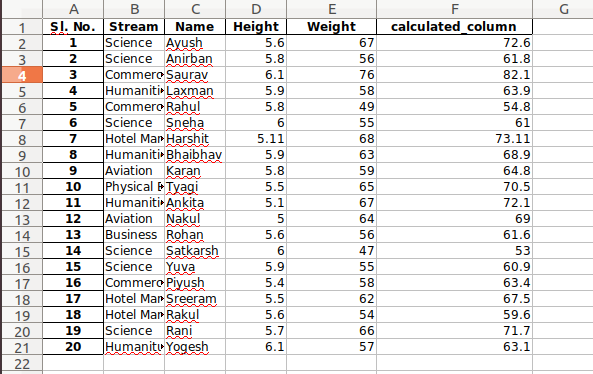My Personal Notes arrow_drop_up